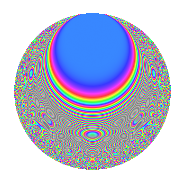# Properties

 Label 935.2.eLevel 935 Weight 2 Character orbit e Rep. character $$\chi_{935}(254,\cdot)$$ Character field $$\Q$$ Dimension 88 Newform subspaces 1 Sturm bound 216 Trace bound 0

# Related objects

## Defining parameters

 Level: $$N$$ = $$935 = 5 \cdot 11 \cdot 17$$ Weight: $$k$$ = $$2$$ Character orbit: $$[\chi]$$ = 935.e (of order $$2$$ and degree $$1$$) Character conductor: $$\operatorname{cond}(\chi)$$ = $$85$$ Character field: $$\Q$$ Newform subspaces: $$1$$ Sturm bound: $$216$$ Trace bound: $$0$$

## Dimensions

The following table gives the dimensions of various subspaces of $$M_{2}(935, [\chi])$$.

Total New Old
Modular forms 112 88 24
Cusp forms 104 88 16
Eisenstein series 8 0 8

## Trace form

 $$88q - 76q^{4} + 80q^{9} + O(q^{10})$$ $$88q - 76q^{4} + 80q^{9} + 12q^{15} + 60q^{16} - 16q^{19} - 24q^{21} + 4q^{25} + 48q^{26} - 36q^{30} - 48q^{34} + 4q^{35} + 12q^{36} + 80q^{49} + 80q^{50} + 28q^{51} - 72q^{59} - 120q^{60} + 4q^{64} + 16q^{66} - 72q^{69} + 24q^{70} - 8q^{76} + 40q^{81} + 32q^{84} + 18q^{85} - 48q^{86} + 48q^{89} + 144q^{94} + O(q^{100})$$

## Decomposition of $$S_{2}^{\mathrm{new}}(935, [\chi])$$ into newform subspaces

Label Dim. $$A$$ Field CM Traces $q$-expansion
$$a_2$$ $$a_3$$ $$a_5$$ $$a_7$$
935.2.e.a $$88$$ $$7.466$$ None $$0$$ $$0$$ $$0$$ $$0$$

## Decomposition of $$S_{2}^{\mathrm{old}}(935, [\chi])$$ into lower level spaces

$$S_{2}^{\mathrm{old}}(935, [\chi]) \cong$$ $$S_{2}^{\mathrm{new}}(85, [\chi])$$$$^{\oplus 2}$$